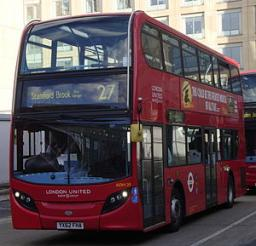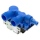# Average speed

When the bus stops at bus stops driving average speed is 45 km/h. If it did not stop it drive at speed 54 km/h. How many minutes of every hour it spend at stops?

Correct result:

t =  10 min

#### Solution:

45•1 = 54•(1-t/60)

54t = 540

t = 10

Our simple equation calculator calculates it.We would be pleased if you find an error in the word problem, spelling mistakes, or inaccuracies and send it to us. Thank you!Tips to related online calculators
Need help calculate sum, simplify or multiply fractions? Try our fraction calculator.
Do you have a linear equation or system of equations and looking for its solution? Or do you have quadratic equation?
Do you want to convert velocity (speed) units?
Do you want to convert time units like minutes to seconds?

## Next similar math problems:

• GardensThe area of the square garden is 3/4 of the area of the triangular garden with sides of 80 m, 50 m, 50 m. How many meters of the fence do we need to fence a square garden?
• Equilateral coneWe pour so much water into a container that has the shape of an equilateral cone, the base of which has a radius r = 6 cm, that one-third of the volume of the cone is filled. How high will the water reach if we turn the cone upside down?
• CoinsThe money - coins are minted from the hardest bronze, which contains copper and tin in a ratio of 41: 9. How much copper and tin are in 2kg of bronze money?How many times does the surface of a sphere decrease if we reduce its radius twice?
• Age of familyThe age of father and son is in the ratio 10: 3. The age of the father and daughter is in ratio 5: 2. How old are a father and a son if the daughter is 20?
• The number 72The number 72 increase by 25%. By how much % will you have to reduce the number you created to get the number 72 again?
• Interior anglesCalculate the interior angles of a triangle that are in the ratio 2: 3: 4.
• LibraryNew books were purchased for the library. Five-eighths were professional books, one-fifth were encyclopedias, and 231 books were dictionaries. How many professional books were there?
• The angles ratioThe angles in the ABC triangle are in the ratio 1: 2: 3. find the sizes of the angles and determine what kind of a triangle it is.
• Triangular prismThe base of the perpendicular triangular prism is a rectangular triangle with a hypotenuse of 10 cm and one leg of 8 cm. The prism height is 75% of the perimeter of the base. Calculate the volume and surface of the prism.
• Water containerThe cube-shaped container is filled to two-thirds of its height. If we pour 18 liters, it will be filled to three-fifths of the height. What is the volume of the whole container?
• Ratio of volumesIf the heights of two cylindrical drums are in the ratio 7:8 and their base radii are in the ratio 4:3. What is the ratio of their volumes?
• Castle towerThe castle tower has a cone-shaped roof with a diameter of 10 meters and a height of 8 meters. Calculate how much m² of coverage is needed to cover it if we must add one-third for the overlap.
• Inlet minus outletWater flows into the tank through two pipes and flows out of the third pipe spontaneously. The pipe A itself would fill the tank in 3 hours, the pipe B itself would fille in 4 hours, and the pipe C itself would flow out in 12 hours. How long will the tank
• Collection of stampsJano, Rado, and Fero have created a collection of stamps in a ratio of 5: 6: 9. Two of them had 429 stamps together. How many stamps did their shared collection have?
• PupilsThere are 32 pupils in the classroom, and girls are two-thirds more than boys. a) How many percents are more girls than boys? Round the result to a whole percentage. b) How many are boys in the class? c) Find the ratio of boys and girls in the class. Writ
• Two friendsPeter can do all his work himself in 6 hours. Martin can do the same work himself in 8 hours. Peter worked first and then replaced by Martin. Whole work was done in 6.5 hours. Calculate how long Peter worked before replaced by Martin.